• Lectures
• Code
• Notebooks
• Community

# The Income Fluctuation Problem¶

## Contents¶

In addition to what’s in Anaconda, this lecture will need the following libraries:

In :
!pip install --upgrade quantecon
!pip install interpolation


## Overview¶

Next, we study an optimal savings problem for an infinitely lived consumer—the “common ancestor” described in [LS18], section 1.3.

This is an essential sub-problem for many representative macroeconomic models

It is related to the decision problem in the stochastic optimal growth model and yet differs in important ways.

For example, the choice problem for the agent includes an additive income term that leads to an occasionally binding constraint.

Our presentation of the model will be relatively brief.

• For further details on economic intuition, implication and models, see [LS18].
• Proofs of all mathematical results stated below can be found in this paper.

To solve the model we will use Euler equation based time iteration, similar to this lecture.

This method turns out to be globally convergent under mild assumptions, even when utility is unbounded (both above and below).

We’ll need the following imports:

In :
import numpy as np
from quantecon.optimize import brent_max, brentq
from interpolation import interp
from numba import njit
import matplotlib.pyplot as plt
%matplotlib inline
from quantecon import MarkovChain


Other useful references include [Dea91], [DH10], [Kuh13], [Rab02], [Rei09] and [SE77].

## The Optimal Savings Problem¶

Let’s write down the model and then discuss how to solve it.

### Set-Up¶

Consider a household that chooses a state-contingent consumption plan $\{c_t\}_{t \geq 0}$ to maximize

$$\mathbb{E} \, \sum_{t=0}^{\infty} \beta^t u(c_t)$$

subject to

$$c_t + a_{t+1} \leq Ra_t + z_t, \qquad c_t \geq 0, \qquad a_t \geq -b \qquad t = 0, 1, \ldots \tag{1}$$

Here

• $\beta \in (0,1)$ is the discount factor
• $a_t$ is asset holdings at time $t$, with ad-hoc borrowing constraint $a_t \geq -b$
• $c_t$ is consumption
• $z_t$ is non-capital income (wages, unemployment compensation, etc.)
• $R := 1 + r$, where $r > 0$ is the interest rate on savings

Non-capital income $\{z_t\}$ is assumed to be a Markov process taking values in $Z\subset (0,\infty)$ with stochastic kernel $\Pi$.

This means that $\Pi(z, B)$ is the probability that $z_{t+1} \in B$ given $z_t = z$.

The expectation of $f(z_{t+1})$ given $z_t = z$ is written as

$$\int f( \acute z) \, \Pi(z, d \acute z)$$

We further assume that

1. $r > 0$ and $\beta R < 1$
2. $u$ is smooth, strictly increasing and strictly concave with $\lim_{c \to 0} u'(c) = \infty$ and $\lim_{c \to \infty} u'(c) = 0$

The asset space is $[-b, \infty)$ and the state is the pair $(a,z) \in S := [-b,\infty) \times Z$.

A feasible consumption path from $(a,z) \in S$ is a consumption sequence $\{c_t\}$ such that $\{c_t\}$ and its induced asset path $\{a_t\}$ satisfy

1. $(a_0, z_0) = (a, z)$
2. the feasibility constraints in (1), and
3. measurability of $c_t$ w.r.t. the filtration generated by $\{z_1, \ldots, z_t\}$

The meaning of the third point is just that consumption at time $t$ can only be a function of outcomes that have already been observed.

### Value Function and Euler Equation¶

The value function $V \colon S \to \mathbb{R}$ is defined by

$$V(a, z) := \sup \, \mathbb{E} \left\{ \sum_{t=0}^{\infty} \beta^t u(c_t) \right\} \tag{2}$$

where the supremum is overall feasible consumption paths from $(a,z)$.

An optimal consumption path from $(a,z)$ is a feasible consumption path from $(a,z)$ that attains the supremum in (2).

To pin down such paths we can use a version of the Euler equation, which in the present setting is

$$u' (c_t) \geq \beta R \, \mathbb{E}_t [ u'(c_{t+1}) ] \tag{3}$$

and

$$u' (c_t) = \beta R \, \mathbb{E}_t [ u'(c_{t+1}) ] \quad \text{whenever } c_t < Ra_t + z_t + b \tag{4}$$

In essence, this says that the natural “arbitrage” relation $u' (c_t) = \beta R \, \mathbb{E}_t [ u'(c_{t+1}) ]$ holds when the choice of current consumption is interior.

Interiority means that $c_t$ is strictly less than its upper bound $Ra_t + z_t + b$.

(The lower boundary case $c_t = 0$ never arises at the optimum because $u'(0) = \infty$)

When $c_t$ does hit the upper bound $Ra_t + z_t + b$, the strict inequality $u' (c_t) > \beta R \, \mathbb{E}_t [ u'(c_{t+1}) ]$ can occur because $c_t$ cannot increase sufficiently to attain equality.

With some thought and effort, one can show that (3) and (4) are equivalent to

$$u' (c_t) = \max \left\{ \beta R \, \mathbb{E}_t [ u'(c_{t+1}) ] \,,\; u'(Ra_t + z_t + b) \right\} \tag{5}$$

### Optimality Results¶

Given our assumptions, it is known that

1. For each $(a,z) \in S$, a unique optimal consumption path from $(a,z)$ exists
2. This path is the unique feasible path from $(a,z)$ satisfying the Euler equality (5) and the transversality condition

$$\lim_{t \to \infty} \beta^t \, \mathbb{E} \, [ u'(c_t) a_{t+1} ] = 0 \tag{6}$$

Moreover, there exists an optimal consumption function $\sigma^* \colon S \to [0, \infty)$ such that the path from $(a,z)$ generated by

$$(a_0, z_0) = (a, z), \quad z_{t+1} \sim \Pi(z_t, dy), \quad c_t = \sigma^*(a_t, z_t) \quad \text{and} \quad a_{t+1} = R a_t + z_t - c_t$$

satisfies both (5) and (6), and hence is the unique optimal path from $(a,z)$.

In summary, to solve the optimization problem, we need to compute $\sigma^*$.

## Computation¶

There are two standard ways to solve for $\sigma^*$

1. Time iteration (TI) using the Euler equality
2. Value function iteration (VFI)

Let’s look at these in turn.

### Time Iteration¶

We can rewrite (5) to make it a statement about functions rather than random variables.

In particular, consider the functional equation

$$u' \circ \sigma \, (a, z) = \max \left\{ \gamma \int u' \circ \sigma \, \{R a + z - c(a, z), \, \acute z\} \, \Pi(z,d \acute z) \, , \; u'(Ra + z + b) \right\} \tag{7}$$

where $\gamma := \beta R$ and $u' \circ c(s) := u'(c(s))$.

Equation (7) is a functional equation in $\sigma$.

In order to identify a solution, let $\mathscr{C}$ be the set of candidate consumption functions $\sigma \colon S \to \mathbb R$ such that

• each $\sigma \in \mathscr{C}$ is continuous and (weakly) increasing
• $\min Z \leq c(a,z) \leq Ra + z + b$ for all $(a,z) \in S$

In addition, let $K \colon \mathscr{C} \to \mathscr{C}$ be defined as follows.

For given $\sigma \in \mathscr{C}$, the value $K \sigma (a,z)$ is the unique $t \in J(a,z)$ that solves

$$u'(t) = \max \left\{ \gamma \int u' \circ \sigma \, \{R a + z - t, \, \acute z\} \, \Pi(z,d \acute z) \, , \; u'(Ra + z + b) \right\} \tag{8}$$

where

$$J(a,z) := \{t \in \mathbb{R} \,:\, \min Z \leq t \leq Ra+ z + b\} \tag{9}$$

We refer to $K$ as Coleman’s policy function operator [Col90].

It is known that

• $K$ is a contraction mapping on $\mathscr{C}$ under the metric
$$\rho(c, d) := \| \, u' \circ \sigma_1 - u' \circ \sigma_2 \, \| := \sup_{s \in S} | \, u'(\sigma_1(s)) - u'(\sigma_2(s)) \, | \qquad \quad (\sigma_1, \sigma_2 \in \mathscr{C})$$
• The metric $\rho$ is complete on $\mathscr{C}$
• Convergence in $\rho$ implies uniform convergence on compacts

In consequence, $K$ has a unique fixed point $\sigma^* \in \mathscr{C}$ and $K^n c \to \sigma^*$ as $n \to \infty$ for any $\sigma \in \mathscr{C}$.

By the definition of $K$, the fixed points of $K$ in $\mathscr{C}$ coincide with the solutions to (7) in $\mathscr{C}$.

In particular, it can be shown that the path $\{c_t\}$ generated from $(a_0,z_0) \in S$ using policy function $\sigma^*$ is the unique optimal path from $(a_0,z_0) \in S$.

TL;DR The unique optimal policy can be computed by picking any $\sigma \in \mathscr{C}$ and iterating with the operator $K$ defined in (8).

### Value Function Iteration¶

The Bellman operator for this problem is given by

$$Tv(a, z) = \max_{0 \leq \sigma \leq Ra + z + b} \left\{ u(c) + \beta \int v(Ra + z - \sigma, \acute z) \Pi(z, d \acute z) \right\} \tag{10}$$

We have to be careful with VFI (i.e., iterating with $T$) in this setting because $u$ is not assumed to be bounded

• In fact typically unbounded both above and below — e.g. $u(c) = \log c$.
• In which case, the standard DP theory does not apply.
• $T^n v$ is not guaranteed to converge to the value function for arbitrary continuous bounded $v$.

Nonetheless, we can always try the popular strategy “iterate and hope”.

We can then check the outcome by comparing with that produced by TI.

The latter is known to converge, as described above.

### Implementation¶

First, we build a class called ConsumerProblem that stores the model primitives.

In :
class ConsumerProblem:
"""
A class that stores primitives for the income fluctuation problem. The
income process is assumed to be a finite state Markov chain.
"""
def __init__(self,
r=0.01,                        # Interest rate
β=0.96,                        # Discount factor
Π=((0.6, 0.4),
(0.05, 0.95)),              # Markov matrix for z_t
z_vals=(0.5, 1.0),             # State space of z_t
b=0,                           # Borrowing constraint
grid_max=16,
grid_size=50,
u=np.log,                      # Utility function
du=njit(lambda x: 1/x)):       # Derivative of utility

self.u, self.du = u, du
self.r, self.R = r, 1 + r
self.β, self.b = β, b
self.Π, self.z_vals = np.array(Π), tuple(z_vals)
self.asset_grid = np.linspace(-b, grid_max, grid_size)


The function operator_factory returns the operator K as specified above

In :
def operator_factory(cp):
"""
A function factory for building operator K.

Here cp is an instance of ConsumerProblem.
"""
# Simplify names, set up arrays
R, Π, β, u, b, du = cp.R, cp.Π, cp.β, cp.u, cp.b, cp.du
asset_grid, z_vals = cp.asset_grid, cp.z_vals
γ = R * β

@njit
def euler_diff(c, a, z, i_z, σ):
"""
The difference of the left-hand side and the right-hand side
of the Euler Equation.
"""
lhs = du(c)
expectation = 0
for i in range(len(z_vals)):
expectation += du(interp(asset_grid, σ[:, i], R * a + z - c)) \
* Π[i_z, i]
rhs = max(γ * expectation, du(R * a + z + b))

return lhs - rhs

@njit
def K(σ):
"""
The operator K.

Iteration with this operator corresponds to time iteration on the
Euler equation.  Computes and returns the updated consumption policy
σ.  The array σ is replaced with a function cf that implements
univariate linear interpolation over the asset grid for each
possible value of z.
"""
σ_new = np.empty_like(σ)
for i_a in range(len(asset_grid)):
a = asset_grid[i_a]
for i_z in range(len(z_vals)):
z = z_vals[i_z]
c_star = brentq(euler_diff, 1e-8, R * a + z + b, \
args=(a, z, i_z, σ)).root
σ_new[i_a, i_z] = c_star

return σ_new

return K


K uses linear interpolation along the asset grid to approximate the value and consumption functions.

To solve for the optimal policy function, we will write a function solve_model to iterate and find the optimal $\sigma$.

In :
def solve_model(cp,
tol=1e-4,
max_iter=1000,
verbose=True,
print_skip=25):

"""
Solves for the optimal policy using time iteration

* cp is an instance of ConsumerProblem
"""

u, β, b, R = cp.u, cp.β, cp.b, cp.R
asset_grid, z_vals = cp.asset_grid, cp.z_vals

# Initial guess of σ
σ = np.empty((len(asset_grid), len(z_vals)))
for i_a, a in enumerate(asset_grid):
for i_z, z in enumerate(z_vals):
c_max = R * a + z + b
σ[i_a, i_z] = c_max

K = operator_factory(cp)

i = 0
error = tol + 1

while i < max_iter and error > tol:
σ_new = K(σ)
error = np.max(np.abs(σ - σ_new))
i += 1
if verbose and i % print_skip == 0:
print(f"Error at iteration {i} is {error}.")
σ = σ_new

if i == max_iter:
print("Failed to converge!")

if verbose and i < max_iter:
print(f"\nConverged in {i} iterations.")

return σ_new


Plotting the result using the default parameters of the ConsumerProblem class

In :
cp = ConsumerProblem()
σ_star = solve_model(cp)

fig, ax = plt.subplots(figsize=(10, 6))
ax.plot(cp.asset_grid, σ_star[:, 0], label='$\sigma^*$')
ax.set(xlabel='asset level', ylabel='optimal consumption')
ax.legend()
plt.show()

Error at iteration 25 is 0.007773142982545167.

Converged in 41 iterations.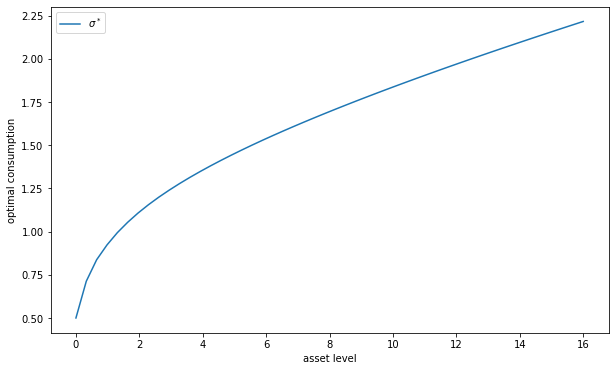The following exercises walk you through several applications where policy functions are computed.

## Exercises¶

### Exercise 1¶

Next, let’s consider how the interest rate affects consumption.

Reproduce the following figure, which shows (approximately) optimal consumption policies for different interest rates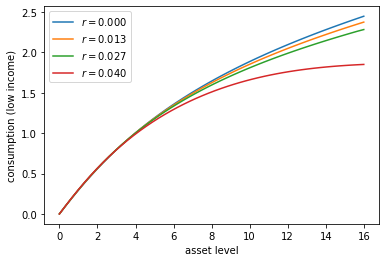• Other than r, all parameters are at their default values.
• r steps through np.linspace(0, 0.04, 4).
• Consumption is plotted against assets for income shock fixed at the smallest value.

The figure shows that higher interest rates boost savings and hence suppress consumption.

### Exercise 2¶

Now let’s consider the long run asset levels held by households.

We’ll take r = 0.03 and otherwise use default parameters.

The following figure is a 45 degree diagram showing the law of motion for assets when consumption is optimal

In :
m = ConsumerProblem(r=0.03, grid_max=4)
K = operator_factory(m)

σ_star = solve_model(m, verbose=False)
a = m.asset_grid
R, z_vals = m.R, m.z_vals

fig, ax = plt.subplots(figsize=(10, 8))
ax.plot(a, R * a + z_vals - σ_star[:, 0], label='Low income')
ax.plot(a, R * a + z_vals - σ_star[:, 1], label='High income')
ax.plot(a, a, 'k--')
ax.set(xlabel='Current assets',
ylabel='Next period assets',
xlim=(0, 4), ylim=(0, 4))
ax.legend()
plt.show()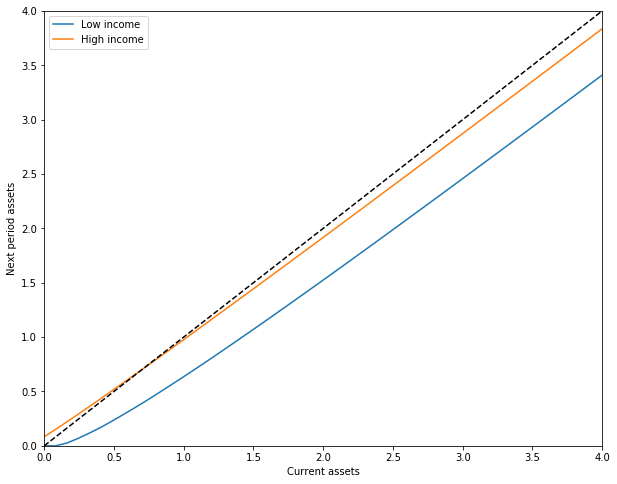The blue line and orange line represent the function

$$a' = h(a, z) := R a + z - \sigma^*(a, z)$$

when income $z$ takes its high and low values respectively.

The dashed line is the 45 degree line.

We can see from the figure that the dynamics will be stable — assets do not diverge.

In fact there is a unique stationary distribution of assets that we can calculate by simulation

• Can be proved via theorem 2 of [HP92].
• Represents the long run dispersion of assets across households when households have idiosyncratic shocks.

Ergodicity is valid here, so stationary probabilities can be calculated by averaging over a single long time series.

Hence to approximate the stationary distribution we can simulate a long time series for assets and histogram, as in the following figure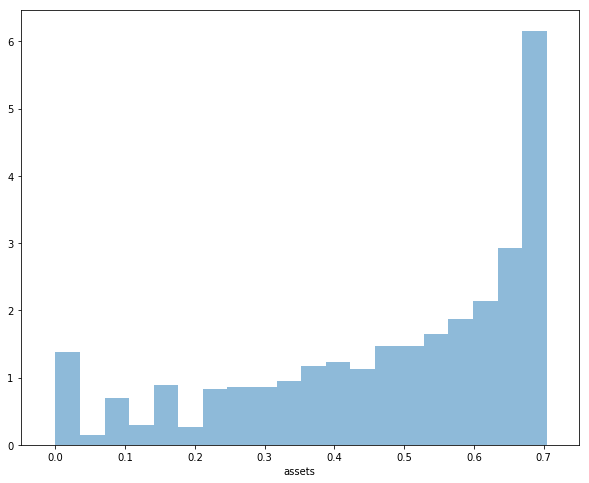• Parameters are as discussed above.
• The histogram in the figure used a single time series $\{a_t\}$ of length 500,000.
• Given the length of this time series, the initial condition $(a_0, z_0)$ will not matter.
• You might find it helpful to use the MarkovChain class from quantecon.

### Exercise 3¶

Following on from exercises 1 and 2, let’s look at how savings and aggregate asset holdings vary with the interest rate

• Note: [LS18] section 18.6 can be consulted for more background on the topic treated in this exercise.

For a given parameterization of the model, the mean of the stationary distribution can be interpreted as aggregate capital in an economy with a unit mass of ex-ante identical households facing idiosyncratic shocks.

Let’s look at how this measure of aggregate capital varies with the interest rate and borrowing constraint.

The next figure plots aggregate capital against the interest rate for b in (1, 3)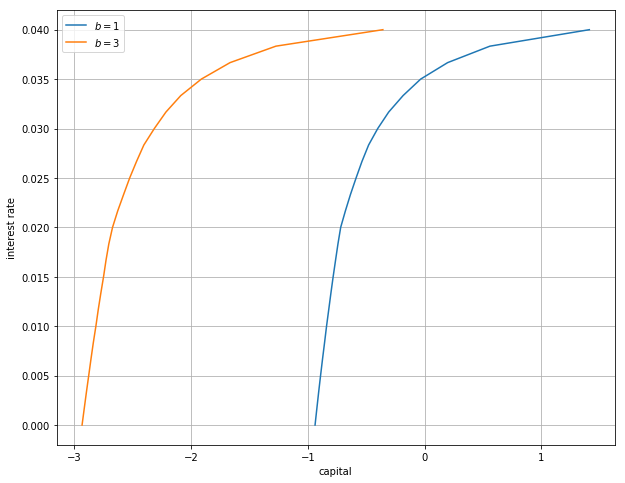As is traditional, the price (interest rate) is on the vertical axis.

The horizontal axis is aggregate capital computed as the mean of the stationary distribution.

Exercise 3 is to replicate the figure, making use of code from previous exercises.

Try to explain why the measure of aggregate capital is equal to $-b$ when $r=0$ for both cases shown here.

## Solutions¶

### Exercise 1¶

In :
r_vals = np.linspace(0, 0.04, 4)

fig, ax = plt.subplots(figsize=(10, 8))
for r_val in r_vals:
cp = ConsumerProblem(r=r_val)
σ_star = solve_model(cp, verbose=False)
ax.plot(cp.asset_grid, σ_star[:, 0], label=f'$r = {r_val:.3f}$')

ax.set(xlabel='asset level', ylabel='consumption (low income)')
ax.legend()
plt.show()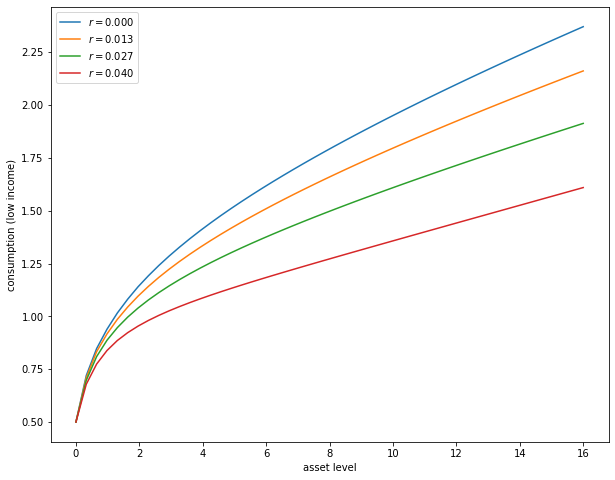### Exercise 2¶

In :
def compute_asset_series(cp, T=500000, verbose=False):
"""
Simulates a time series of length T for assets, given optimal
savings behavior.

cp is an instance of ConsumerProblem
"""
Π, z_vals, R = cp.Π, cp.z_vals, cp.R  # Simplify names
mc = MarkovChain(Π)
σ_star = solve_model(cp, verbose=False)
cf = lambda a, i_z: interp(cp.asset_grid, σ_star[:, i_z], a)
a = np.zeros(T+1)
z_seq = mc.simulate(T)
for t in range(T):
i_z = z_seq[t]
a[t+1] = R * a[t] + z_vals[i_z] - cf(a[t], i_z)
return a

cp = ConsumerProblem(r=0.03, grid_max=4)
a = compute_asset_series(cp)

fig, ax = plt.subplots(figsize=(10, 8))
ax.hist(a, bins=20, alpha=0.5, density=True)
ax.set(xlabel='assets', xlim=(-0.05, 0.75))
plt.show()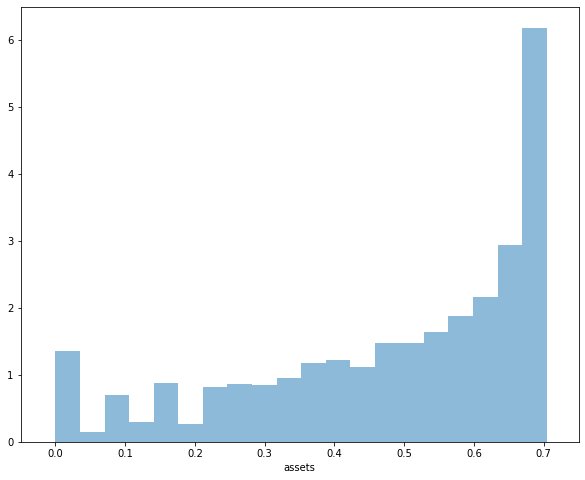### Exercise 3¶

In :
M = 25
r_vals = np.linspace(0, 0.04, M)
fig, ax = plt.subplots(figsize=(10, 8))

for b in (1, 3):
asset_mean = []
for r_val in r_vals:
cp = ConsumerProblem(r=r_val, b=b)
mean = np.mean(compute_asset_series(cp, T=250000))
asset_mean.append(mean)
ax.plot(asset_mean, r_vals, label=f'$b = {b:d}$')
print(f"Finished iteration b = {b:d}")

ax.set(xlabel='capital', ylabel='interest rate')
ax.grid()
ax.legend()
plt.show()

Finished iteration b = 1
Finished iteration b = 3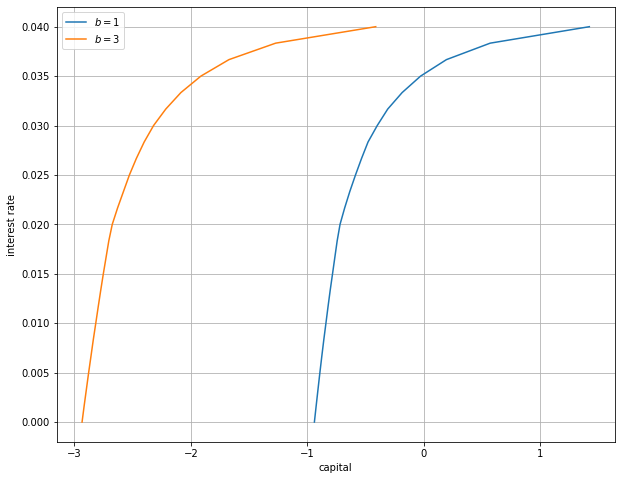• Share page